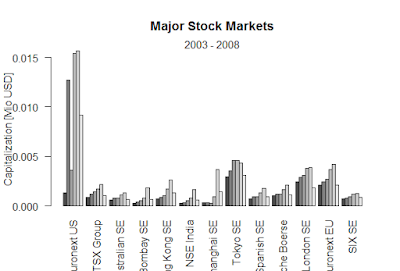Showing posts with label One sample t-test. Show all posts
Showing posts with label One sample t-test. Show all posts

## What is the basic structure of the hypothesis test?

The most common being observation following a normal distribution.
The ᕼo(Null) and Ⱨ(alternative hypothesis are specified) mostly null specifies a particular value of a parameter.
Under the general assumption, we take Ho is true, the distribution of the test statistic is known.

Given the distribution and value of the test statistic and the form of Ⱨ, we can calculate the P-value of the test.
Based on p-value and pre-specified level of significance, we make decision
Fail to reject Ho
Reject the Ho

### One sample t-test in R

we have taken 9 random sample
t.test(x = apt_crisp\$weight, mu = 16, alternative = c("less"), conf.level = 0.95)
> apt_crisp = data.frame(weight = c(15.5, 16.2, 16.1, 15.8, 15.6, 16.0, 15.8, 15.9, 16.2))
> x_bar = mean(apt_crisp\$weight)
> s = sd(apt_crisp\$weight)
> mu_0 = 16
> n = 9
> t = (x_bar - mu_0) / (s / sqrt(n))
> t
 -1.2
> pt(t, df = n - 1)
 0.1322336
data:  apt_crisp\$weight
t = -1.2, df = 8, p-value = 0.1322
alternative hypothesis: true mean is less than 16
95 percent confidence interval:
-Inf 16.05496
sample estimates:
mean of x
15.9

> apt_test_results = t.test(apt_crisp\$weight, mu = 16,
+ alternative = c("two.sided"), conf.level = 0.95)
> names(apt_test_results)
 "statistic"   "parameter"   "p.value"
 "conf.int"    "estimate"    "null.value"
 "stderr"      "alternative" "method"
 "data.name"
> qt(0.975, df = 8)
 2.306004
apt_test_results\$conf.int
 15.70783 16.09217
attr(,"conf.level")
 0.95
> c(mean(apt_crisp\$weight) - qt(0.975, df = 8) * sd(apt_crisp\$weight) / sqrt(9),
+   mean(apt_crisp\$weight) + qt(0.975, df = 8) * sd(apt_crisp\$weight) / sqrt(9))
 15.70783 16.09217

### Black-Scholes formula-R

Black-Scholes formula-R > BlackScholes <- function(TypeFlag = c("c", "p"), S, X, Time, r, b, sigma) { TypeFla...